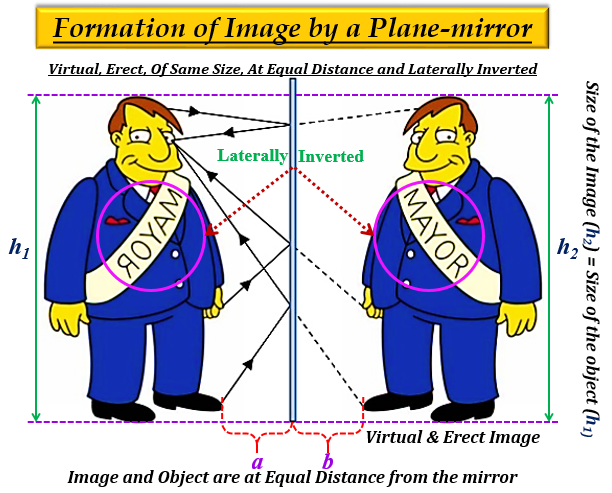# If an object is placed at a distance of 10 cm in from of a plane mirror, how far would it be from its image?

It is one of the characteristics of a plane mirror that, the distance of the plane mirror from the object is equal to its distance from the image.

Therefore, given distance between object and mirror = 10cm.

Distance between mirror and image = 10cm.

Thus, the distance between object and image = 10 + 10 = 20cm.

Hence, if an object is placed at a distance of 10 cm in from of a plane mirror, then it would be 20cm far away from its image

Explanation with the image is posted for reference only.

The distance of the image formed by the plane mirror is equal to the distance of the object from the mirror. It is one of the characteristics of the plane mirror.

Other characteristics of the image formed by a plane mirror are virtual, erect (upright), of the same size, and at the same distance.

Plane mirrors are used in kaleidoscopes, shaving mirrors, dentists' mirror, torch lights, etc.Updated on: 10-Oct-2022

1K+ Views